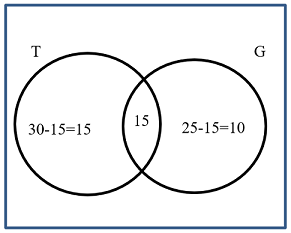## Pricing Table Particle

• Basic
• Standard Compliant Channels
• $50 • Completely synergize resource taxing relationships via premier market • 1 GB of space • Support at$25/hour
• Standard Compliant Channels
• $100 • Completely synergize resource taxing relationships via premier market • 10 GB of space • Support at$15/hour
• Platinum
• Standard Compliant Channels
• $250 • Completely synergize resource taxing relationships via premier market • 30 GB of space • Support at$5/hour

### Search Our Site

Sets
Mathematics
Topic One

A set is a group of objects of the same kind and these objects in a set are called the elements or members of a set. A set is well-defined when all its members can be listed. Sets are represented by a Venn diagram in which a rectangle is used to represent a universal set, U, and circles inside the rectangle are used to represent subsets.

Examples of well-defined sets

1. A = the set of even numbers between 1 and 19

"$= \{2, 4, 6, 8, 10, 12, 14, 16, 18\}$"

2. B = the set of multiples of 5 between 8 and 36

"$= \{10, 15, 20, 25, 30, 35 \}$"

"$\{ \}$" means ‘the set of’

# Finite and Infinite Sets

A finite set is one in which it is possible to list and count all the members of the set.

Example

"$M = \{\text {months of the year}\}$"

"$= \{ \text{January, February, March, April, May, June, July, August, September, October, November, December} \}$"

An infinite set is one in which it is not possible to list and count all the members of the set.

Example

"$E = \{ \text {odd numbers greater than 1} \}$"

# Equal and Equivalent Sets

Two sets are equal if they both have the same members.

Example

If, "$N= \{40, 60, 100\}$"

and, "$M = \{100, 60, 40 \}$"

then, "$N=M$", that is both sets are equal.

Note: The order in which the members of a set are written does not matter.

Two sets are equivalent if they have the same number of elements.

Example

If, "$O = \{ 2, 4, 6, 8, 10 \}$"
and "$P = \{ 10, 14, 16, 20, 24 \}$"
then, "$n(O)= n(P)= 5$", that is, sets "$O$" and "$P$" are equivalent.

# Empty Sets

An empty set is a set which has no members.

Example

"$Q = \{\}$"

# Subsets

A set M is a subset of a set X, if all the elements of M are in the larger set X.

Example

If, "$X = \{ 3, 5, 7, 9, 11, 15, 17, 19 \}$"
and, "$M = \{ 11, 17, 19 \}$"
then, M is a subset of X.
That is, "$M \subset X$" (where "$\subset$" means ‘is a subset of’).

# Universal Sets

The universal set is the set from which all the elements being examined are members. The universal set is denoted by the symbol "$\bigcup$".

Example

If, "$\bigcup = \{ 3, 5, 7, 9, 11, 15, 17, 19 \}$"
and, "$M = \{ 11, 17, 19 \}$"
then M is a subset of "$\bigcup$".
Then "$\bigcup$" is the universal set as M is derived from "$\bigcup$".

# Intersection of Sets

The intersection of two sets is the listing of elements that are in both sets.  An intersection of A and B is shown in the Venn diagram below as "$A \cap B$", where "$\cap$" means ‘intersect’.

Venn Diagrams

As stated earlier, sets are represented by a Venn diagram in which a rectangle is used to represent a universal set, U, and circles inside the rectangle are used to represent subsets.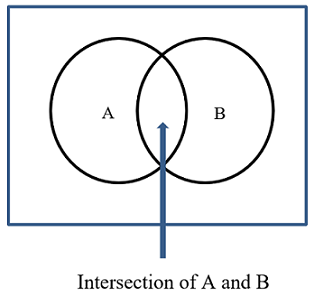Example

If "$\bigcup = \{ 2, 4, 6, 8, 10, 12, 14, 16, 18, 20 \}$"
"$A = \{ 4, 6, 8, 10, 12 \}$"
"$B = \{ 2, 4, 5, 10, 12, 14 \}$"Then, A intersects B, "$A \bigcap B = \{ 4, 10, 12 \}$"

The numbers 16, 18 and 20 are outside of both A and B.

# Union of Sets

The union of two sets A and B is the set of elements that are in A or B, or both. The Venn diagram below shows A ⋃ B.Example

If, "$\bigcup = \{ 2, 4, 6, 8, 10, 12, 14, 16, 18, 20 \}$"
"$A = \{ 4, 6, 8, 10, 12 \}$"
"$B = \{ 2, 10, 12, 14 \}$"

Then, A union B, "$A \bigcup B = \{ 2, 4, 6, 8, 10, 12, 14 \}$"

Note:

"$\left ( A \bigcup B \right )’ = \{16, 18, 20 \}$"
and,
"$\left ( A \bigcup B \right )’ = A’ \bigcap B’$" which we will prove momentarily.

# Complement

The complement of a set A, written A’, is the set of all the members of the universal set, which are not elements of the set A.

Example

If, "$\bigcup = \{ 3, 5, 7, 9, 11, 13, 15, 17, 19 \}$"
and, "$A = \{ 5, 11, 17, 19 \}$"
then, "$A’ = \{ 3, 7, 9, 13, 15 \}$"
where, A’ is “the complement of A.

Example- Using Venn diagram

A represents all the elements that are in the universal set "$\bigcup$" and A’ represents all of the numbers in "$\bigcup$" that are not in A.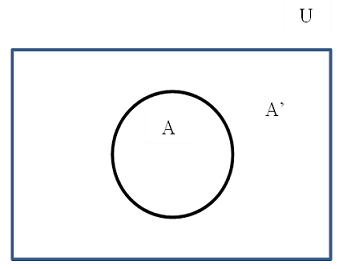Using the example above, below is a Venn diagram showing A’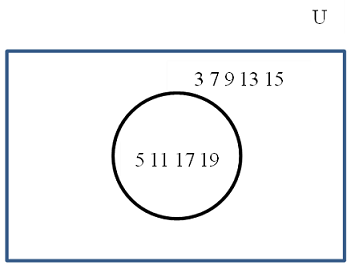Example:

If, "$\bigcup = \{ 2, 4, 6, 8, 10, 12, 14, 16, 18, 20 \}$"
"$A = \{ 4, 6, 8, 10, 12 \}$"
"$B = \{ 2, 10, 12, 14 \}$"

Solution:

As we saw above, A union B, "$A \bigcup B = \{ 2, 4, 6, 8, 10, 12, 14 \}$"

and "$\left ( A \bigcup B \right )’ = \{ 16, 18, 20 \}$" which is what is in "$\bigcup$" that is not in A and B.  Also,

"$A’ = \{ 14, 16, 18, 20 \}$" and "$B’ = \{ 4, 6, 8, 16, 18, 20 \}$".

"$A’ \bigcap B’ = \{ 16, 18, 20 \}$"

So
"$\left ( A \bigcup B \right )’ = A’ \bigcup B’$"

Also, "$A \bigcap B = \{ 10, 12 \}$".# Three Sets

We can also have a case where there are three sets such as follows:

"$\bigcup = \{ 1, 2, 3, 4, 5, 6. 7, 8, 9, 10, 12, 14 \}$",

"$A = \{ 1, 2, 3, 4, 5, 6, 7 \}$",

"$B = \{ 2, 4, 6, 7, 8, 9 \}$", and

"$C = \{ 3, 5, 6, 7, 9 \}$".

The set A contains the numbers 1, 2, 3, 4, 5, 6 and 7. The numbers 6 and 7 are in all three sets so it is all three circles.  Also, A shares 2, 4, 6 and 7 with B, so these will go in the rest of the overlap of these two sets. In addition, A shares 3, 5, 6 and 7 with C, so this will go in the rest of the overlap area with C. The set B shares 6, 7 and 9 with C.  The remaining elements, being in this case just the numbers 1 and 8, go into the only-A part of A's circle and only-B part of B’s circle respectively.  There are three numbers which are not in any of the named sets.  These numbers are 10, 12 and 14 and these are placed outside of these three circles.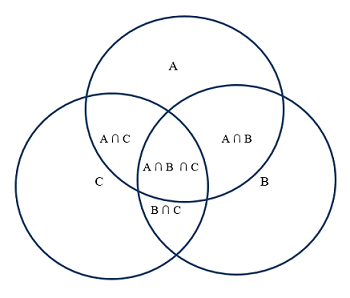# Number of Elements in named Subsets

This involves determining the number of elements to be named in the subsets of two intersecting sets, given the number of elements in some of the other subsets.

Example 1

In a class of 40 students, 28 studied accounts, 32 studied science and 22 students studied both Accounts and Science.  Determine the number of students who studied:
(i) accounts only
(ii) science only.

Solution

Let, "$A = \{ \text{students who studied accounts} \}$"
"$S = \{ \text{students who studied science} \}$"

Seeing that 22 students studied both accounts and science, it is common to both subjects and so they are in the intersection.  If we take these 22 students from students who studied accounts and science, we will be able to get the amount of students who studied accounts alone and science alone.

Given information:

"$n(A) = 28$"
"$n(S) = 32$"
"$n(A \bigcap S) = 22$"

Using a Venn diagram: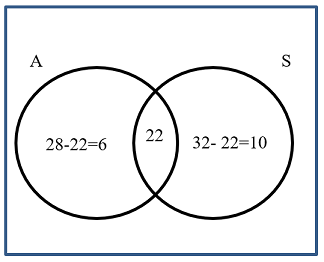Therefore, 6 students studied accounts alone and 10 students studied science alone.

Example 2

At a sporting club, there are 50 members where 30 members play tennis and 25 members play golf while 10 members play neither tennis nor golf. Calculate the number of members who play:

(i) both tennis and golf
(ii tennis only
(iii) golf only.

Solution

Let, "$T = \{ \text{members who play tennis} \}$"
"$G = \{ \text{members who play golf} \}$"
Given information:
Universal set "$n \left( \bigcup \right) = 50$"
"$n(T) = 30$"
"$n(G) = 25$"
"$n(T \bigcup G) ’ = 10$"

Let x represent the number of members who like both tennis and golf which is
"$n(T \bigcap G) = x$"

Then, the number of members who like tennis only is represented by,
"$n(T \bigcap G’) = 30 –x$"

Also, the number of members who like golf  only is represented by,
"$n(G \bigcap T’) = 25 –x$"

Using a Venn diagram: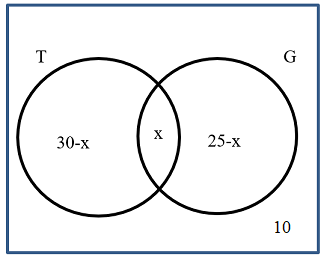Solving:

(i) Members who like both tennis and golf
"$n( \bigcup ) = 30 – x + x + 25 – x + 10$"
since "$n(U) = 50$", then
"$50 = 30 – x + x + 25 – x + 10$"

"$50 = 30 + 25 + 10 –x + x – x$"

"$50 = 65 - x$"

"$-65+50 = – x$"

"$-15 = – x$"
"$\left( -15 = -x \right)$" times by -1 gives

"$15 = x$"

"$x = 15$"

that is, x=15 members

Therefore, 15 students play both tennis and golf.

(ii) Members who like tennis only
"$n(T \bigcap G’) = 30 –x$" members
"$= 30 – 15$"
"$= 15$"

So, 15 students play tennis only.

(iii) Members who like golf only
"$n(T’ \bigcap G) = 25 –x$" members
"$= 25 – 15$"
"$= 10$"

So, 10 students play golf only.